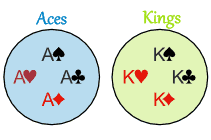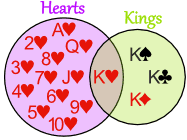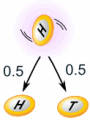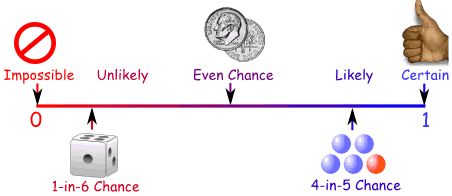×#### Thank you for registering.

One of our academic counsellors will contact you within 1 working day.

Click to Chat

1800-1023-196

+91-120-4616500

CART 0

• 0

MY CART (5)

Use Coupon: CART20 and get 20% off on all online Study Material

ITEM
DETAILS
MRP
DISCOUNT
FINAL PRICE
Total Price: Rs.

There are no items in this cart.
Continue Shopping```Basics of Probability

Table of Content

Definition of Experiment

Random Experiment

Bernoulli Trial

​Sample Space

Complement of An Event

Mutually Exclusive Events

Equally Likely Events

Exhaustive Events

Mutually Exclusive and Exhaustive Events

Simple Events

Independent Events

Dependent Events

Definition of ExperimentIn probability theory, an experiment or a trial is any procedure that can be infinitely repeated and has either a well-defined set of possible outcomes or no certain outcomes. Whenever an experiment is conducted, it only results in one outcome though this outcome may be included in various events. Any particular performance of a random experiment is called a trial.

An experiment is a set of processes terms, which are carried out under stipulated conditions to study the phenomena associated with it. Broadly, there can be two types of experiments:

(i) Experiments with definite outcome: These types of experiments are certain in nature. Outcomes of experiments are known in advance.

(ii) Experiments with indefinite outcome: These types of experiments are uncertain in nature and it is not possible to predict the outcome with certainty.

Random Experiment

An experiment whose all possible outcomes (results) are known in advance, but the result of any specific performance cannot be predicted before completion of the experiment is called a random experiment. In other words, an experiment is said to be random if it has more than one possible outcome. On the other hand, an experiment is said to be deterministic if it has only one possible outcome. A random experiment that has exactly two possible outcomes is known as a Bernoulli trial.

Illustration:

Consider the following experiments.

(i) Tossing a coin

(ii) Rolling a die

(iii) Drawing a card from a pack of well-shuffled pack of 52 cards.

Solution:

All the experiments have more than one possible outcome. All outcomes are known in advance. For example in the first case, when we toss a coin it is known that the outcome will be either a head or a tail. Likewise, rolling of a die results in one of the numbers among 1 to 6. Hence all these experiments are random experiments.

Bernoulli Trial

In many experiments there are only two outcomes. For instance:

Flip a coin.

Take a penalty shot on goal.

Test a randomly selected circuit to see whether it is defective.

Roll a die and determine whether it is a 6 or not.

Such an experiment which has only two possible outcomes is called a Bernoulli trial. The two outcomes are usually the success and the failure. In such a trial, the probability of each outcome is the same in each trial and the outcome of each trial is independent of the outcomes of the other trials. If the probability of success is p, the probability of failure is 1 - p. The flip of a coin is a Bernoulli trial (where the probability of both success and failure is 0.5), as is the roll of a die (where success might be arbitrarily defined as rolling a six and failure as rolling any other number, with the probability of success being 0.167 and the probability of failure 0.833).

​Sample Space

The set of all possible outcomes of an experiment (or trial) is called the sample space. It is usually denoted by s.

A die is thrown. The upper face can show any of the six numbers (1, 2, 3, 4, 5, 6). Due to this, we get a sample space consisting of 6 elements.

Activity

Sample Space

Rolling a die

There will be 6 outcomes in the sample space:
{1, 2, 3, 4, 5, 6}

A card is drawn from a well-shuffled pack of cards. We get a sample space consisting of 52 elements.

Drawing a card from a standard deck

There will be 52 cards in the sample space:
{Spades: 2,3,4,5,6,7,8,9,10, ace, jack queen, king,
Clubs:  2,3,4,5,6,7,8,9,10, ace, jack, queen, king,
Diamonds:  2,3,4,5,6,7,8,9,10, ace, jack, queen, king,
Hearts:  2,3,4,5,6,7,8,9,10, ace, jack, queen, king}

For example, for the experiment of tossing a coin, the sample space is a set S = {head, tail}. For the experiment of tossing two coins, the sample space is S = {head - head, head - tail, tail - head, tail - tail}.Example: A spinner has four equal sectors coloured yellow, blue, green and red., the sample space in this case is given by:Sample Space

{Yellow, Green, Blue, Red}

Event:

The appearance of a particular outcome which might also be present in the sample space is called an event. In fact, any subset of the sample space is called an event. Choosing an ace from a pack of well-shuffled cards is an event. This event occurs 4 times in a pack of card i.e. in sample space. Observing an even number when a dice is thrown is an event. This event occurs three times in the sample space of 6 elements. So, event is a subset of sample space.

Complement of An Event

Let S be the sample space and A be some event, then the set of all out comes which are in S but not in A is called the complete of event A. It is denoted by A’ , A- or Ac.

Illustration:

In rolling a die event A is described as numbers

A = {2, 4, 6}

Ac = {1, 3, 5}

Rolling of a dice

Experiment:  Tossing a coin

Event

A

Complement

A'

The coin shows tails.

Experiment:  Drawing a card

Event

A

The card is black.

Complement

A'

The card is red.

Illustration: A pair of dice is rolled.  What is the probability of not rolling doubles?

Solution: This is a question involving the complement of an event. Hence, in this case, rolling doubles means the probability of getting the same number on both the die. There are 6 ways of getting the same number on both the die.

So P (doubles) = 6/36

Hence, P (not doubles) = 1-6/36

= 5/6.

There are several types of events. Now we discuss each of them in detail:

Mutually Exclusive Events

Let the two events be A and B. If occurrence of event A excludes the possibility of occurrence of B and vice-versa, then we say that A and B are mutually exclusive event i.e. they cannot occur simultaneously. It (i.e. mutual exclusion) can happen only when the sets of two events have no common point.

Example:

In rolling a die, event A is described as appearance of an even number and event B is described as appearance of an odd number.

A = {2, 4, 6}

B = {1, 3, 5}

Clearly if one says that A has occurred we will instantly conclude that B cannot occur (in that trial of course). So,

A ∩ B = ΦExamples:
A pair of dice is rolled.  The events of rolling a 6 and of rolling a double have the outcome (3, 3) in common.  These two events are NOT mutually exclusive.

A pair of dice is rolled.  The events of rolling a 9 and of rolling a double have NO outcomes in common.  These two events ARE mutually exclusive.

If A and B are mutually exclusive events,
P (A or B) = P (A) + P (B)

Example: A pair of dice is rolled.  What is the probability that the sum of the numbers rolled is either 7 or 11?

Solution: We first list the outcomes that have the sum as 7:

(1, 6), (2, 5), (3, 4), (4, 3), (5, 2), (6, 1)
P (7) = 6/36

Two outcomes have a sum of 11:
(5, 6), (6, 5)
P(11) = 2/36

Since the sum of numbers cannot be 7 and 11 at the same time, so these events are mutually exclusive.

P(7 or 11) = P(7) + P(11) = 6/36 + 2/36 = 8/36 = 2/9.

For more, you may refer the video

Equally Likely Events

Two or more events are said to be equally likely when there is no reason to prefer one event over other i.e. they have equal (not same) number of points in their sets.

Illustration:

In rolling a die event A is described as number showing less than 4 and event B is described as number appearing greater than 3.

A = {1, 2, 3}

B = {4, 5, 6}

Clearly A and B have equal number of points in the sample space and hence are equally likely.

Exhaustive Events

If n events A1, A2, ........., An related to any particular sample space are such that if we take union of the sets of all the n events, sample space is formed. i.e.

A1∪A2 ∪ A3 ∪ ...............  ∪An = S

Illustration:

In rolling a die three events are described as follows

A1 : even number appears

A2 : less than 4 appears

A3 : greater than 4 appears

Hence A1 : {2, 4, 6}

A2 : {1, 2, 3}

A3 : {5, 6}

A1 ∪A ∪ A3 = {1, 2, 3, 4, 5, 6} which is the sample space for the experiment of rolling a die.

Mutually Exclusive and Exhaustive Events

Events A1, A2, .......... An are said to be mutually exclusive and exhaustive if they satisfy the condition for mutual exclusion and exhaustiveness both.

i.e.    A1 ∪ A2 ∪ A3 ............ ∪ An = S

and   Ai ∩ Aj = Φ, where i = 1, 2, ........., n

j = 1, 2, ........... n and i ≠ j.

i.e. mutually exclusive and exhaustive events are those events of which union is equal to sample space and occurrence of any one of them excludes the possibility of occurrence of all others.

Illustration:

In rolling a die, events A1, A2, A3 and A4 are described as follows

A1 : Number less then 2 occurs.

A2 : 2 occurs

A3 : odd number greater that 1 occur.

A4 : even number greater than 2 occur.

A1 : {1}

A2 : {2}

A3 : {3, 5}

A4 : {4, 6}

Clearly, A1 υ A2 υ A3 υ A4 = {1, 2, 3, 4, 5, 6} = S

and   A1 ∩ A2 = A1 ∩ A3 = A1 ∩ A4 = A2 ∩ A3 = A2 ∩ A4 = A3 ∩ A4 = Φ

i.e. Ai ∩ Aj = Φ, i ≠ j, i, j = 1, 2, 3, ............ n.

Simple Events

When an event corresponds to a single outcome of the activity, it is often called a simple event.

Example: Drawing the queen of spades from a deck of standard cards.

Probability of this event= 1/52

Independent Events

If the occurrence or nonoccurrence of any event does not affect the sample space of occurrence of the other, then two events are said to independent.Example: You toss a coin and it comes up "Heads" three times ... what is the chance that the next toss will also be a "Head"?

The chance is simply ½ (or 0.5) like any other toss of a coin. The current toss does not depend on what came in the previous toss.

Dependent Events

Dependent events are those events which are affected by other events.

Probability Rules

There are various rules of probability which are very important:

The probability of an event always lies between 0 and 1.

0 ≤P (E) ≤1The sum of all the probabilities in the sample space is 1.

The probability of an event which cannot occur is 0.

The probability of any event which is not in the sample space is zero.

The probability of an event which must occur is 1.

The probability of the sample space is 1.

The probability of an event not occurring is one minus the probability of it occurring.

P (E') = 1 - P (E)

Illustration 1: One hundred identical coins each with probability ‘p’, of showing up heads are tossed once. If 0<p<1 and the probability of showing heads on 50 coins is equal to that of heads showing on 51 coins, then the value of p is

1. 1/2                                                   2. 49/101

3. 50/101                                             4.  51/101

Solution: Let X be the number of coins showing heads. Hence, we assume X to be a binomial variate with parameters n and p where n=100.

Since, P(X = 50) = P(X = 51)

100C50p50 (1-p)50 = 100C51p51(1-p)49

This implies that [100! / 50! 50!] (1-p) = [100! / 51! 49!] p

1-p/ p = 50/51

This gives the value of p = 51/100.

Illustration 2: the probability that at least one of the events A and B occurs is 0.6. If A and B occur simultaneously with probability 0.2, then P(Ac) + P(Bc) is

1. 0.4                                                        2. 0.8

3. 1.2                                                        4. 1.4

5. None of these

Solution: It is given in the question that P(A∪B) = 0.6 and P(A∩B) = 0.2

We know that P(A + B) = P(A) + P(B) – P(AB)

And P(Ac) + P(Bc) = 1- P(A) + 1- P(B)

= 2 - [P(A) + P(B)]

= 2 - [P(A+B) + P(AB)]

= 2 - [0.6+0.2]

= 2 - 0.8 = 1.2

Note: P(A∪B) is written as P(A+B) and P(A∩B) is written as P(AB).

Illustration 3: If (1+3p)/3, (1-p)/4 and (1-2p)/2 are the probabilities of three mutually exclusive events, then the set of all values of p is ….

Solution: Since the probabilities of (1+3p)/3, (1-p)/4 and (1-2p)/2 are given to be mutually exclusive events,

So, (1+3p)/3 + (1-p)/4 + (1-2p)/2 ≤ 1

4 + 12p + 3 - 3p + 6 - 12p ≤ 12

Hence, 13 – 3p ≤ 12

So, p ≥ 1/3

0 ≤ 1+3p/3 ≤ 1

-1/3 ≤ p ≤ 2/3

0 ≤ 1 - p/4 ≤ 1

-3 ≤ p ≤ 1

0 ≤ (1 - 2p)/2 ≤ 1

-1/2 ≤ p ≤ 1/2

-1/3 ≤ p ≤ 1/2

Hence, the value of p lies between -1/3 ≤ p ≤ 1/2.

Free study material for the preparation of IIT JEE is available online at askIITians.com. You may also refer the Model Papers to get an idea about the types of questions asked.

To read more, Buy study materials of Probability comprising study notes, revision notes, video lectures, previous year solved questions etc. Also browse for more study materials on Mathematics here.
```### Course Features

• 731 Video Lectures
• Revision Notes
• Previous Year Papers
• Mind Map
• Study Planner
• NCERT Solutions
• Discussion Forum
• Test paper with Video Solution